# Flow Meter: What They Are & Types of Flow Meters

Contents

## What is a Flow Meter?

A flow meter is a piece of equipment used to measure the flow rate of solids, liquids, or gases. Flow meters can do this linearly, non-linearly, volumetrically, or on a weight basis. Flow meters are also known as flow gauges, flow indicators, or liquid meters.

## Types of Flow Meters

The main types of flow meters include:

1. Mechanical Flow Meters
2. Optical Flow Meters
3. Open Channel Flow Meters

### Mechanical Flow Meters

#### Positive Displacement Flow Meters

These meters measure the flow rate by measuring the volume of the fluid passing through them. The actual process includes entrapment of the fluid into the certain container-like thing so as know its flow rate. This is very much similar to the case wherein we allow water to fill a bucket up to a certain predetermined level, after which it is allowed to flow out.

These flow meters can measure intermittent flows or meager flow rates and are suitable for any liquid irrespective of their viscosity or density. Positive displacement flowmeters can be considered to be robust as they remain unaffected by the turbulence in the pipe.

Nutating disc meter, Reciprocating piston meter, Oscillatory or Rotary piston meter, Bi-rotor type meters like those of Gear meter, Oval gear meter (Figure 1) and Helical gear meter fall under this category.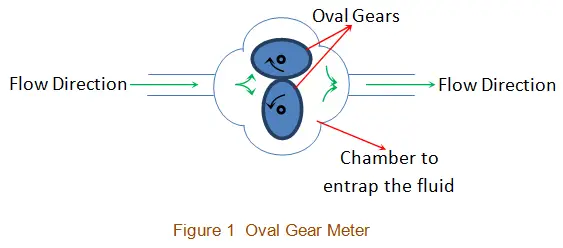#### Mass Flow Meters

These meters provide the user with an estimate of the flow rate by measuring the mass of the substance flowing through them. This kind of weight-oriented flow meters is usually employed in chemical industries wherein the weight-based measurement is essential in comparison to volumetric analysis.

Thermal meters (Figure 2a) and Coriolis flowmeters (Figure 2b) fall under this category. In the case of thermal meters, the fluid flow cools-off the probe, which is pre-heated to a certain degree. The heat loss can be sensed and will be used to determine the rate at which the fluid will be flowing. On the other hand, Coriolis meters work on the principle of Coriolis principle in which the fluid flow through the vibrating tube causes a change in frequency or phase shift or amplitude, which gives a measure of its flow rate.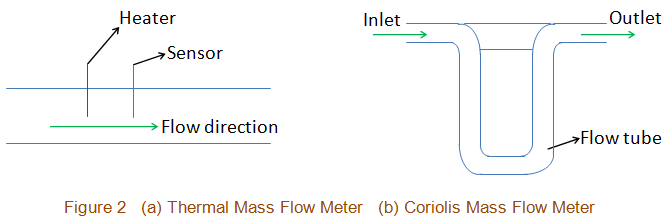#### Differential Pressure Flow Meters

In a differential pressure flow meters, the flow is measured by measuring the drop in pressure as the fluid passes through the obstructions, which are inserted into the path along which it flows. This is because as the flow of the fluid through the pipe increases, there will be more drop in the pressure across the constriction (Figure 3), which can be recorded by the meters. From this, one can compute the flow rate as it will be proportional to the square root of the pressure drop (Bernoulli’s equation).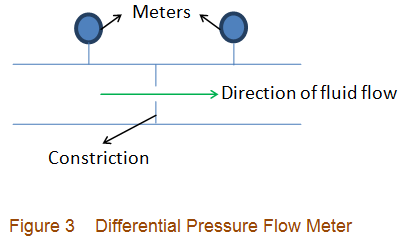Orifice plate meter, Flow nozzle meter, Flow tube meter, Pilot tube meter, Elbow tap meter, Target meter, Dall tube meter, Cone meter, Venturi tube meter, Laminar flow meter, and Variable Area meter (Rotameter) are a few examples of differential pressure flow meters.

#### Velocity Flow Meters

Velocity flow meters estimate the flow rate of the fluids by measuring the velocity of the fluid which flows through them. Here the velocity of the fluid gives a direct measure of its flow rate as they are directly proportional to each other. In these meters, one can measure the velocity in a variety of ways in which the use of the turbine is also one (Figure 4).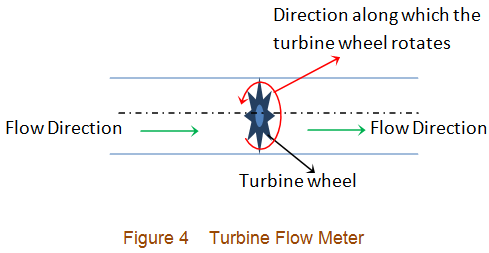Depending on the way used to find out the velocity, we have various kinds of velocity flow meters like Turbine flow meter, Vortex Shedding flow meter, Pitot tube flow meter, Propeller flow meter, Paddle or Pelton wheel flow meter, Single jet flow meter and Multiple jet flow meter.

Flow rate measurement of fluids in hazardous environments, including those in the case of mining demands for the non-intrusive flow meters. SONAR flow meters which are a type of velocity flow meters serve this kind of requirements. Besides, ultrasonic flow meters, as well as electromagnetic flow meters, also form a part of velocity-type flow meters.

### Optical Flow Meters

Optical flow meters work on the principle of optics i.e. they measure the flow rate using light. Usually, they employ a set-up consisting of a laser beam and photodetectors. Here, the particles of the gas flowing through the pipe scatter the laser beam to produce pulses which are picked-up by the receiver (Figure 5). Then, the time between these signals is determined as one would know the distance by which the photodetectors would be separated, which in turn leads to the measurement of the speed of gas.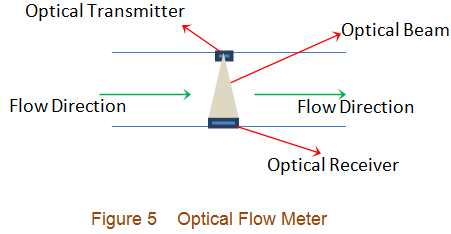As these meters measure the actual speed of the particles constituting the gases, they remain unaffected by the thermal conditions and the variations in gas flow. Hence, they are capable of providing highly accurate flow data even when the environment is most unfavorable, say, for example, when we have high temperature and pressure, high humidity, etc.

### Open Channel Flow Meters

Open channel flow meters are used to measure the flow rate of a fluid whose flow path involves a free surface. Weir meters and Flume meters (Figure 6) are the open channel flow meters which use secondary devices like bubblers or float to measure the depth of the fluid at a particular point. From this depth, the flow rate of the fluid can be obtained.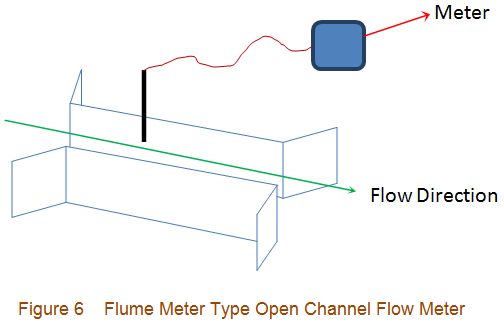On the other hand, in dye-testing based open channel flow measurement case, a certain predetermined amount of dye or salt is used to change the concentration of the flowing stream of the fluid. The resulting dilution gives a measure of flow rate of the fluid. Next, it is to be noted that the precision with which the flow meters need to operate is decided by the application for which they are used. For example, when we want to monitor the flow of water through a pipe in our garden, it would be enough if we use a flow meter which has a lower precision than that which will be used when we need to monitor the flow of an alkali meant for a chemical process. Besides, one more factor which requires to be noted is that the flow meters, when used in conjunction with the flow valves, can perform the controlling actions successfully.

## What is a Water Meter?

A water meter is a type of flow meter which is used to monitor the flow rate of water through a pipe. There are two common approaches to water flow measurement – displacement and velocity. Common displacement designs include oscillating piston and nutating disc meters. Velocity-based designs include single and multi-jet meters and turbine meters.

## Types of Water Meters

Water meters can be classified into various types depending on the mechanism by which they measure the flow of water.

### Gear Type Water Flow Meter

Generally, all residential water meters are of positive displacement type. These can in turn be gear meter- (Figure 1) or oscillating piston or nutating disk meter-type. Here, the water is made to enter into a chamber from which it is let out only when the chamber fills-out.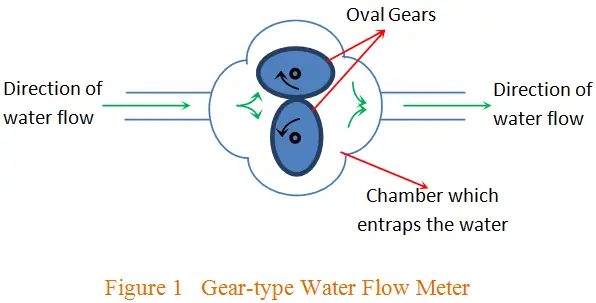By doing so, one can estimate the rate of flow of water. These meters are used when the water flows at a moderately low rate.

### Single Jet Water Meter

Velocity water meters, also known as internal capacity meters, are another category of water flow meters. In these meters, the rate of flow of water is determined by monitoring the speed at which the water flows. The subcategories under this type are jet (single- and multi-jet) and turbine flow meters.

In single-jet meter, a single water-jet impinges on the impeller while in the case of multi-jet meter, more than one jet is impinged on it. However in either cases, the rotational speed of the impeller gives a measure of flow rate of water. On the other hand, the turbine-kind of water meters use a turbine wheel whose speed of rotation determines the flow rate.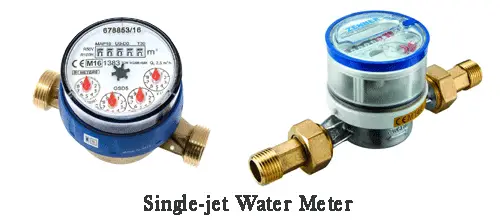Here it is to be noted that jet-type water meters are suitable for low flow rate measurements, while turbine-type flow meters are suitable when flow rates are high. Hence when one has to accomplish both high as well as low flow rate measurements, better choice would be compound-type water meters, which combine both of these categories into a single device.

### Electromagnetic Water Meter

Water meters can also measure the flow rate of water by using Faraday’s law of induction. Such meters are referred to as electromagnetic water meters (Figure 2) and are usually used when one needs to measure unclean or untreated or wastewater.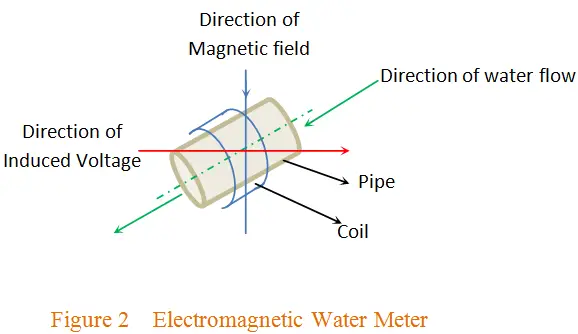Here, the water flowing through the non-magnetic and non-electrical pipe induces a voltage in the meter’s magnetic field. The magnitude of this voltage will be proportional to the magnetic flux density and hence to the velocity of water flowing through the pipe, from which rate of flow of water can be determined.

### Transit Time Type Water Meter

Water meters can also be of an ultrasonic type, in which the rate of water flow is measured by using SONAR techniques. Here the sound waves are sent through the flowing water to measure its velocity. Once the velocity is known, one can determine the associated rate of flow of water as the cross-sectional area of the meter body would be known in prior. These kind of meters can either be of Doppler-type or of Transit-time type.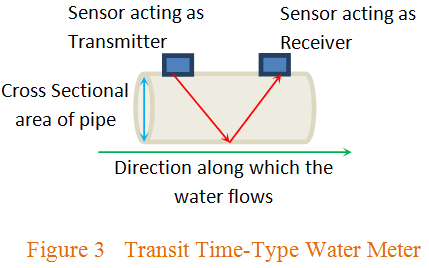## Applications of Water Meter

The applications of water meters include:

1. The water supply departments are the primary users of water meters. This department has these kind of meters installed in every building so as to track the amount of water consumed by them. The intention behind such an act is to bill them accordingly.
2. Large infrastructures employ water meters to ensure proper water flow through each of their sub-structures, free from leakage and breaks.
3. Industries which have cooling as one of the step in their process use water meter to monitor the rate at which the water flows.
4. Water meters are also employed in agricultural industries and labs with an intention of analyzing different properties of water like its salinity, pH level, acidity, etc.
5. Hydroelectric plants which generate electric power using water employ water meter to maintain a controlled amount of water flow through them.
6. Water meters like that of turbine-type are used in fire protection systems.

## Flow Measurement

Flow measurement is a method of measuring how much of a material flows through a particular area of interest (Figure 1). Generally, industrial processes demand for the measurement of three types of ‘materials’ viz., solids, liquids and gases.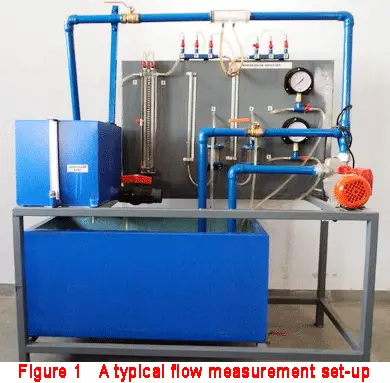### Measurement of Solids

Solids are usually measured on their weight-basis i.e. the flow rate of solids will be expressed as the ratio of mass of the solid per unit time or in terms of weight of the solid per unit time. Thus we have its associated units as kilogram-per-second or gram-per-second or kilogram-per-hour or gram-per-minute or tonnes-per-hour or likewise.

### Measurement of Liquids

Liquids can be either measured in terms of their weight or volume. This means that the flow rate of liquids is expressed as the ratio of weight of the liquid per unit time or volume of the liquid per unit time. Accordingly, we can express their rate in units such as tonnes-per-hour, kilogram-per-second, liters-per-hour, m3-per-minute and so on.

### Measurement of Gases

Gases are usually measured in terms of their volume i.e. the flow rate of gases will be expressed as the ratio of the volume of the gas-flow per unit time. Thus the units in which the flow of gases is measured are m3-per-hour, Nm3-per-hour, etc. One more important factor to be noted while measuring the flow of gases is the fact that the gases are compressible fluids (unlike liquids) due to which their volume varies in accordance with the pressure (and also temperature). In order to account for this factor, usually the values obtained by measuring the gases at the original conditions are converted into normal temperature and pressure (NTP) base or to Standard Temperature and Pressure (STP) base.

### Flow Meters

Flow measurement is accomplished through the use of flow meters (Figure 2), as discussed in detail earlier in this article.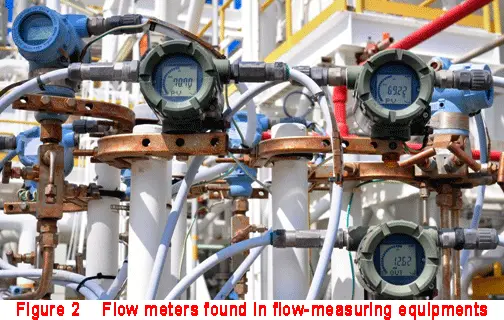These flow meters are of different types depending on the quantity being measured and the principle on which they operate. Differential pressure meter, variable area meter, turbine flow meter, Coriolis meter, gear meter, Woltman meter, single jet meter, multiple jet meter, paddle wheel meter, current meter, Venturi meter, cone meter, linear resistance meter, Vortex flow meter, magnetic flow meter, ultrasonic flow meter, non-contact electromagnetic flow meter, etc are only a few among the numerous types of flow meters in-use.

### Flow Sensors

Sensors are the devices which are used to monitor the change in a particular parameter. Accordingly, flow sensors are the devices which are used to monitor the changes in the flow-rate of a particular state of matter. These are expected to record even the slightest changes in the flow rate and are thus expected to be of high precision.

The existence of numerous kinds of flow meters and flow sensors are a direct consequence of the importance which is attributed to the concept of flow measurement in numerous industrial applications, including the design of automatic control systems.

Want To Learn Faster? 🎓
Get electrical articles delivered to your inbox every week.
No credit card required—it’s 100% free.